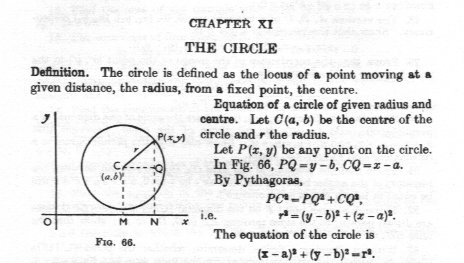# General equation of the circle

## Recommended Posts

How we can derive the general equation of the circle?

X square + Y square + 2gx + 2fy + c = 0

Thanks & Regards,

Prashant S Akerkar

##### Share on other sites

Derive from what?

Another way to view this equation is

(x - f)2 +(y - g)2 = r2

This give a circle, centre (f, g) radius r.

Multiply it out and compare.

##### Share on other sites

You can probably get there from trigonometry. Given a centre position X and Y and radius r, you could integrate tan over 360 degrees...

##### Share on other sites

You don't need trigonometry to obtain this standard result, just good old Pythagoras.##### Share on other sites

Thanks.

1 Is

X square + Y square + 2gx + 2fy + c = 0

and

(x-a) square + (y-b) square = r square

same?

2 What will be the general equation of the semicircle?.

Is it

(x-a) square + (y-b) square = r square / 2 ?

Thanks & Regards,

Prashant S Akerkar

##### Share on other sites

3 hours ago, prashantakerkar said:

1 Is

X square + Y square + 2gx + 2fy + c = 0

and

(x-a) square + (y-b) square = r square

same?

Yes

3 hours ago, prashantakerkar said:

2 What will be the general equation of the semicircle?.

Is it

(x-a) square + (y-b) square = r square / 2 ?

No that is a circle with a smaller radius (70.7% of the original radius)

For a semicircle, you need to define a line through the centre of the circle and add the condition that the included points have to be on one side of the line.

##### Share on other sites

Thanks.

What will be then the general equation of the Semicircle?

Thanks & Regards,

Prashant S Akerkar

##### Share on other sites

27 minutes ago, prashantakerkar said:

Thanks.

What will be then the general equation of the Semicircle?

Thanks & Regards,

Prashant S Akerkar

5 hours ago, prashantakerkar said:

Thanks.

1 Is

X square + Y square + 2gx + 2fy + c = 0

and

(x-a) square + (y-b) square = r square

same?

2 What will be the general equation of the semicircle?.

Is it

(x-a) square + (y-b) square = r square / 2 ?

Thanks & Regards,

Prashant S Akerkar

You need to show you understand more basic algebra before tackling the next stage.

Did you do the algebraic comparison I suggested or just guess?

A semicirircle does not have a single equation.
It is described by the intersection of a circle and a straight line along with some conditions.

##### Share on other sites

Thank you.

Will there be more than one general equations of the Semicircle ?

How to derive the general equation of a Semicircle by drawing a Semicircle figure?

Thanks & Regards,

Prashant S Akerkar

##### Share on other sites

You need to do more than nod wisely when someone tells you something.

Your responses tell me that you are not understanding what we are telling you.

I am not saying this to be nasty, but because you need to think before you answer.

In particular you need to answer the questions we are asking, they are all meant to help.

You are not doing this at the moment.

##### Share on other sites

• 3 weeks later...
On ‎7‎/‎25‎/‎2018 at 5:10 AM, prashantakerkar said:

Thank you.

Will there be more than one general equations of the Semicircle ?

How to derive the general equation of a Semicircle by drawing a Semicircle figure?

Thanks & Regards,

Prashant S Akerkar

What semi-circle?  You have already been told that the equation of a circle with center at (a, b) and radius r is (x- a)^2+ (y- b)^2= r^2.  The equation of the semi-circle consisting of the right half of that circle is (x- a)^2+ (y- b)^2= r^2 with the restriction that x> a.  Similarly, the left half is the same but with x< a, the top is with y> b and the bottom y< b.

## Create an account

Register a new account